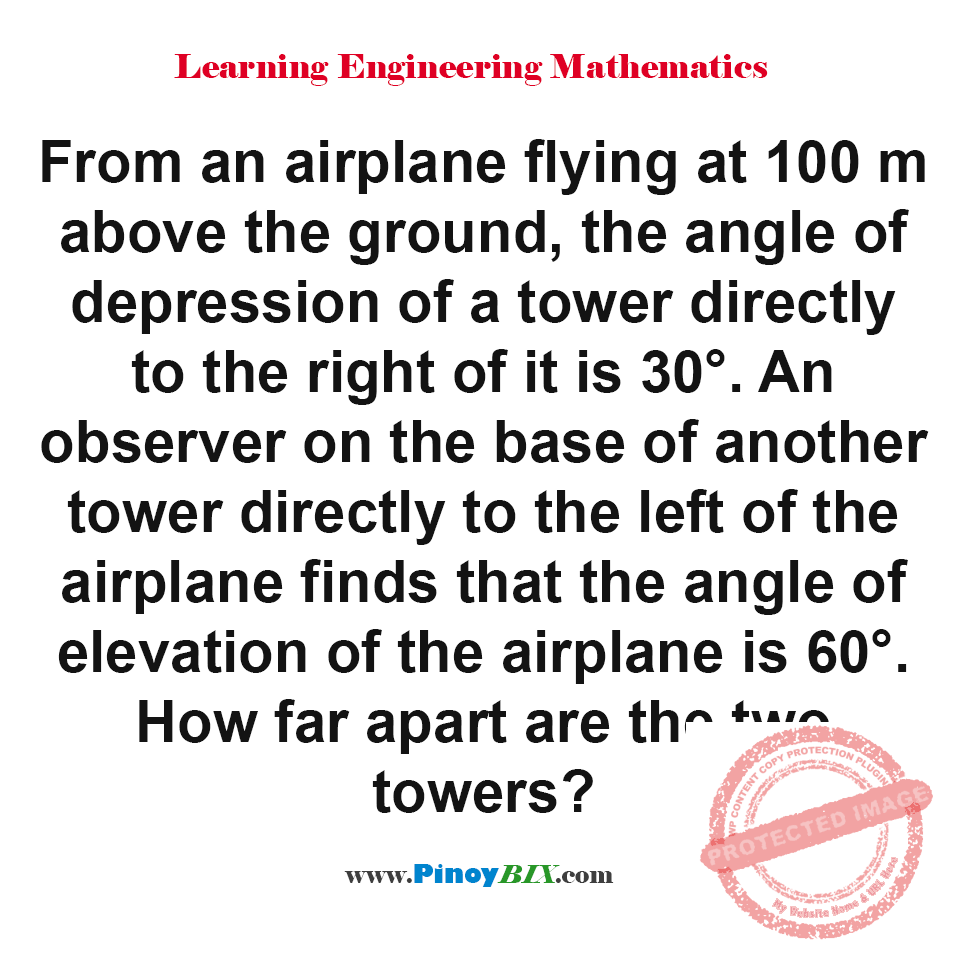Solution: Find the distance between the two towers

(Last Updated On: March 6, 2019)Problem Statement:

From an airplane flying at 100 m above the ground, the angle of depression of a tower directly to the right of it is 30°. An observer on the base of another tower directly to the left of the airplane finds that the angle of elevation of the airplane is 60°. How far apart are the two towers?

The two towers are 230.94 meters apart.

Solution:

Latest Problem Solving in Plane Trigonometry Problems

More Questions in: Plane Trigonometry Problems

Online Questions and Answers in Plane Trigonometry Problems

Solution: Find the distance between the two towers
5 (100%) 1 vote[s]

NEED SUPPORT: LIKE MY PAGE & SHARE